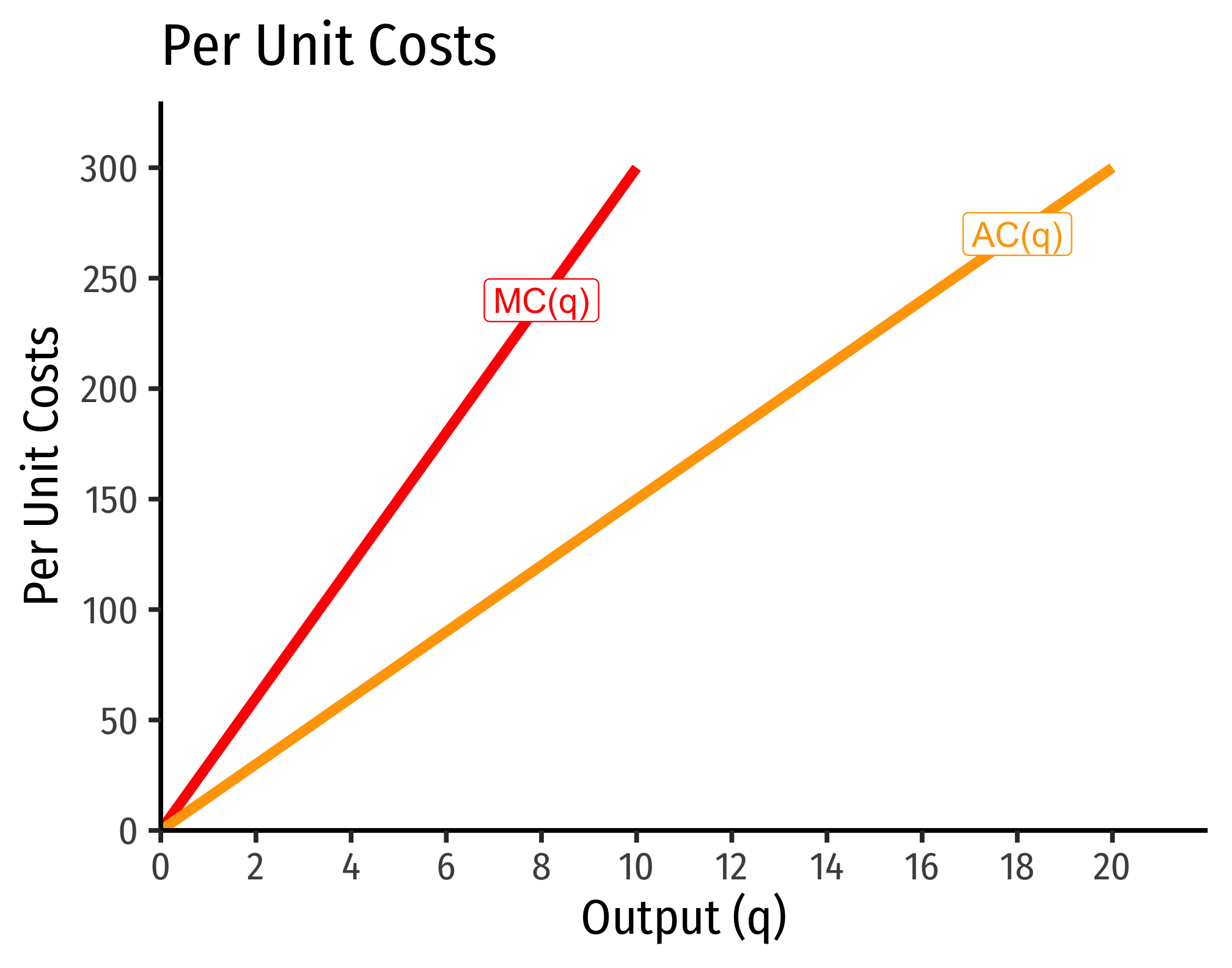# 2.4 — Costs of Production — Class Notes

## Contents

Wednesday, September 23, 2020

## Overview

Today we cover costs before we put them together next class with revenues to solve the firm’s (first stage) profit maximization problem. While it seems we are adding quite a bit, and learning some new math problems with calculating costs, we will practice it more next class, when put together with revenues.

## Appendix

### The Relationship Between Returns to Scale and Costs

There is a direct relationship between a technology’s returns to scaleIncreasing, decreasing, or constant

and its cost structure: the rate at which its total costs increaseAt a decreasing rate, at an increasing rate, or at a constant rate, respectively

and its marginal costs changeDecreasing, increasing, or constant, respectively

. This is easiest to see for a single input, such as our assumptions of the short run (where firms can change $$l$$ but not $$\bar{k})$$:

$q=f(\bar{k},l)$

#### Constant Returns to Scale: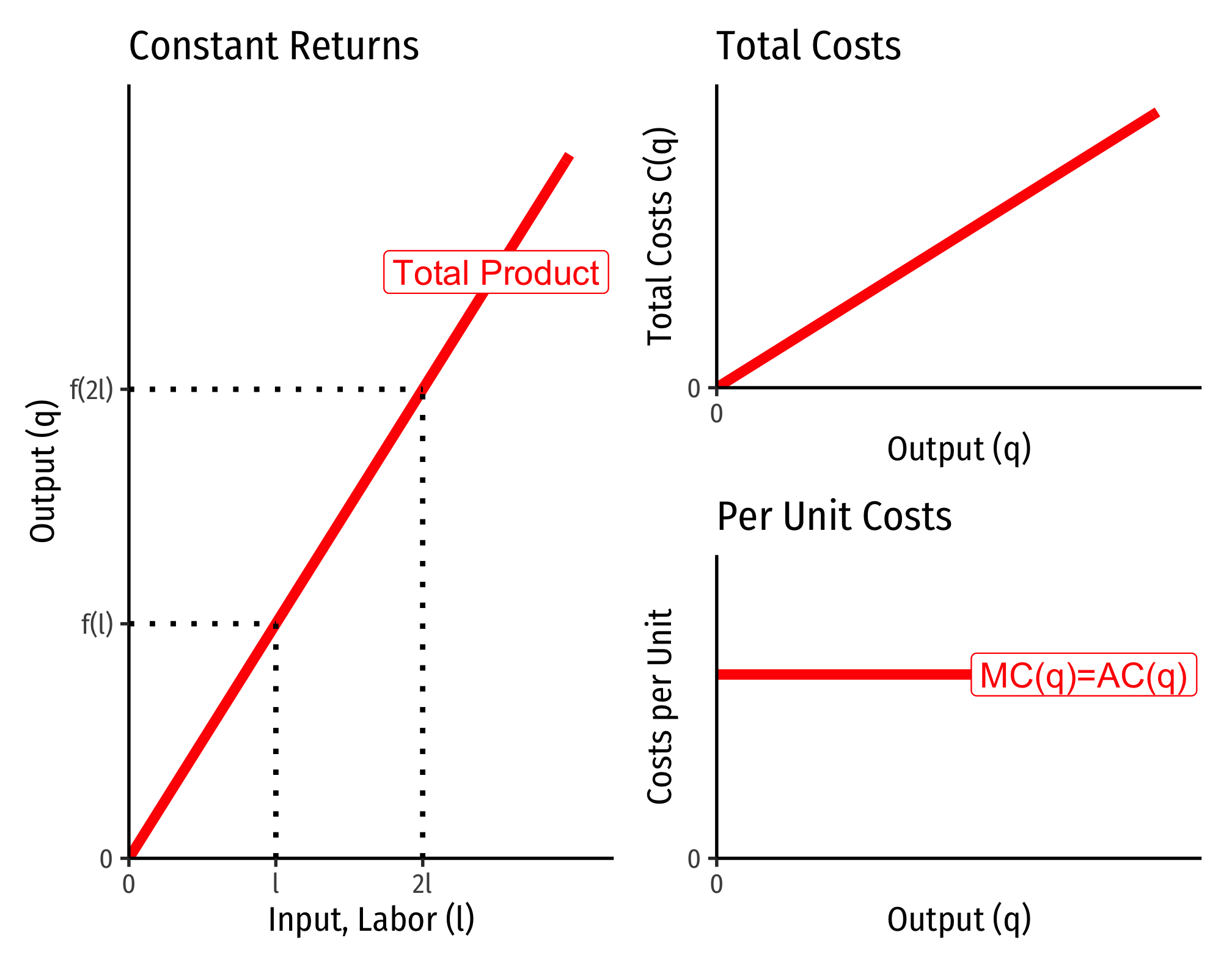#### Decreasing Returns to Scale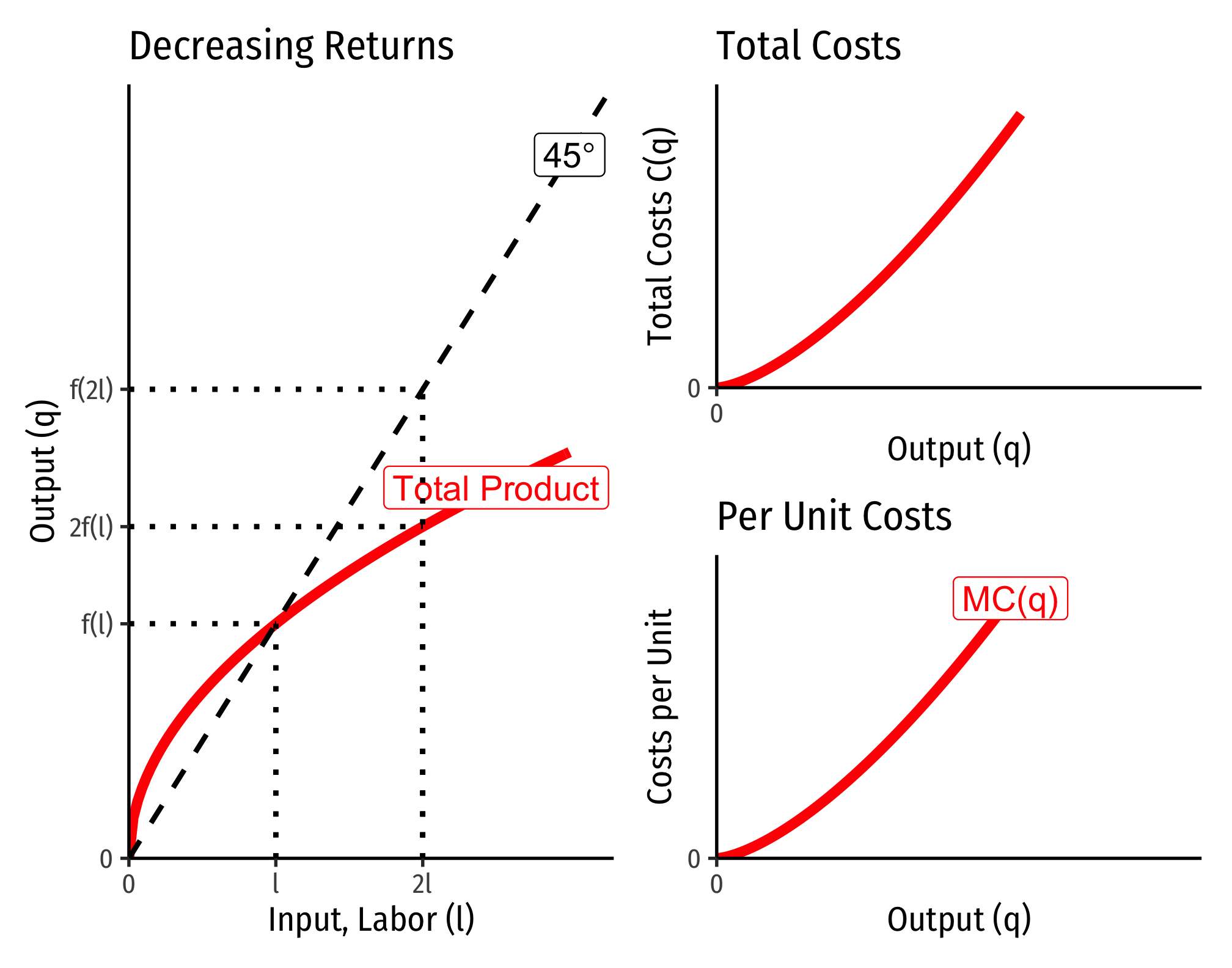#### Increasing Returns to Scale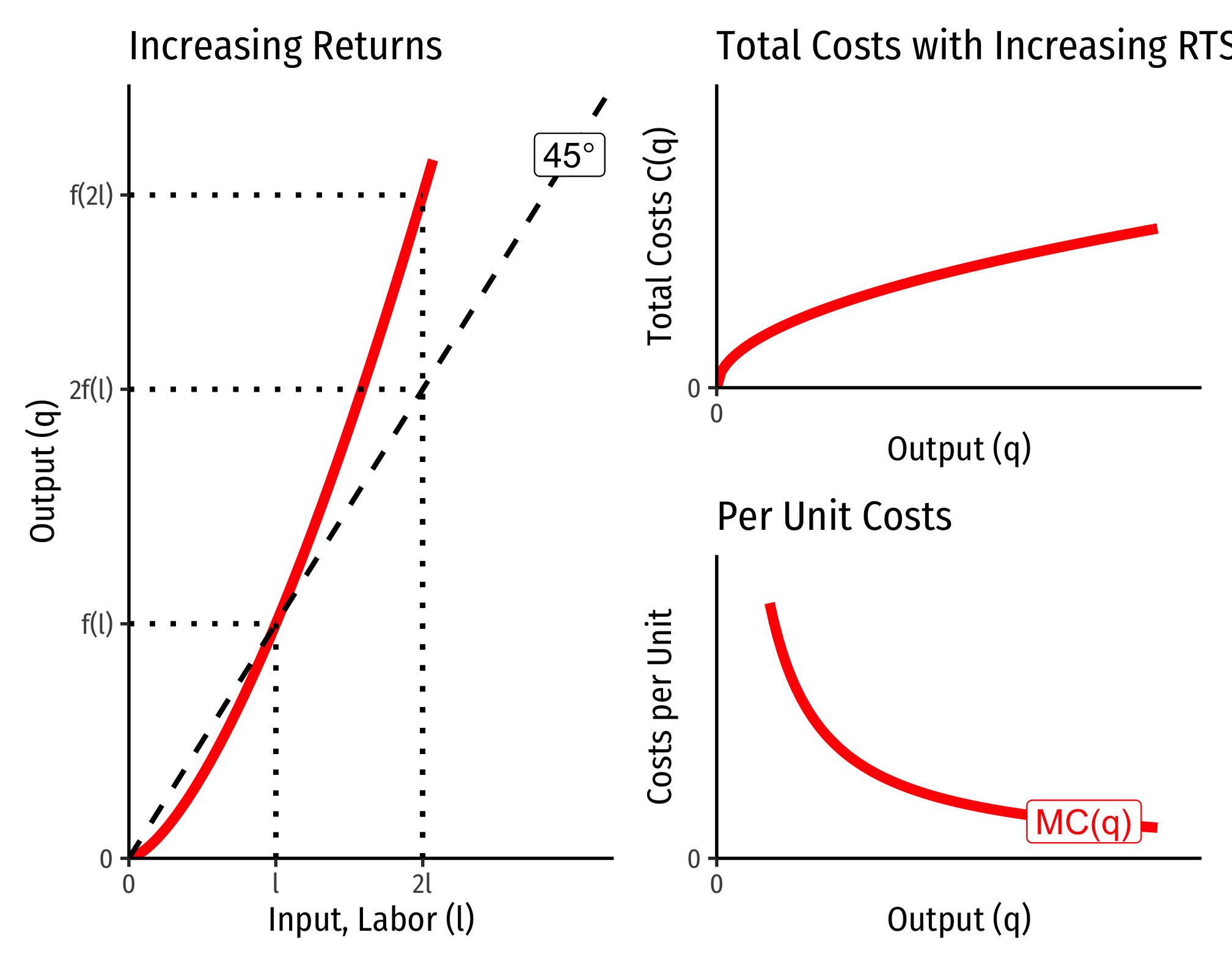### Cobb-Douglas Cost Functions

The total cost function for Cobb-Douglas production functions of the form $q=l^{\alpha}k^{\beta}$ can be shown with some very tedious algebra to be:

$C(w,r,q)=\left[\left(\frac{\alpha}{\beta}\right)^{\frac{\beta}{\alpha+\beta}} + \left(\frac{\alpha}{\beta}\right)^{\frac{-\alpha}{\alpha+\beta}}\right] w^{\frac{\alpha}{\alpha+\beta}} r^{\frac{\beta}{\alpha+\beta}} q^{\frac{1}{\alpha+\beta}}$

If you take the first derivative of this (to get marginal cost), it is:

$\frac{\partial C(w,r,q)}{\partial q}= MC(q) = \frac{1}{\alpha+\beta} \left(w^{\frac{\alpha}{\alpha+\beta}} r^{\frac{\beta}{\alpha+\beta}}\right) q^{\left(\frac{1}{\alpha+\beta}\right)-1}$

How does marginal cost change with increased output? Take the second derivative:

$\frac{\partial^2 C(w,r,q)}{\partial q^2}= \frac{1}{\alpha+\beta} \left(\frac{1}{\alpha+\beta} -1 \right) \left(w^{\frac{\alpha}{\alpha+\beta}} r^{\frac{\beta}{\alpha+\beta}}\right) q^{\left(\frac{1}{\alpha+\beta}\right)-2}$

Three possible cases:

1. If $$\frac{1}{\alpha+\beta} > 1$$, this is positive $$\implies$$ decreasing returns to scale
• Production function exponents $$\alpha+\beta < 1$$
1. If $$\frac{1}{\alpha+\beta} < 1$$, this is negative $$\implies$$ increasing returns to scale
• Production function exponents $$\alpha+\beta > 1$$
1. If $$\frac{1}{\alpha+\beta} = 1$$, this is constant $$\implies$$ constant returns to scale
• Production function exponents$$\alpha+\beta = 1$$

#### Example (Constant Returns)

Let $$q=l^{0.5}k^{0.5}$$.

\begin{align*} C(w,r,q)&=\left[\left(\frac{0.5}{0.5}\right)^{\frac{0.5}{0.5+0.5}} + \left(\frac{0.5}{0.5}\right)^{\frac{-0.5}{0.5+0.5}}\right] w^{\frac{0.5}{0.5+0.5}} r^{\frac{0.5}{0.5+0.5}} q^{\frac{1}{0.5+0.5}}\\ C(w,r,q)&= \left[1^{0.5}+1^{-0.5} \right]w^{0.5}r^{0.5}q^{0.5}\\ C(w,r,q)&= w^{0.5}r^{0.5}q^{1}\\ \end{align*}

Consider input prices of $$w=\9$$ and $$r=\25$$:

\begin{align*}C(w=10,r=20,q)&=9^{0.5}25^{0.5}q \\ & =3*5*q\\ & =15q\\\end{align*}

That is, total costs (at those given input prices, and technology) is equal to 15 times the output level, $$q$$: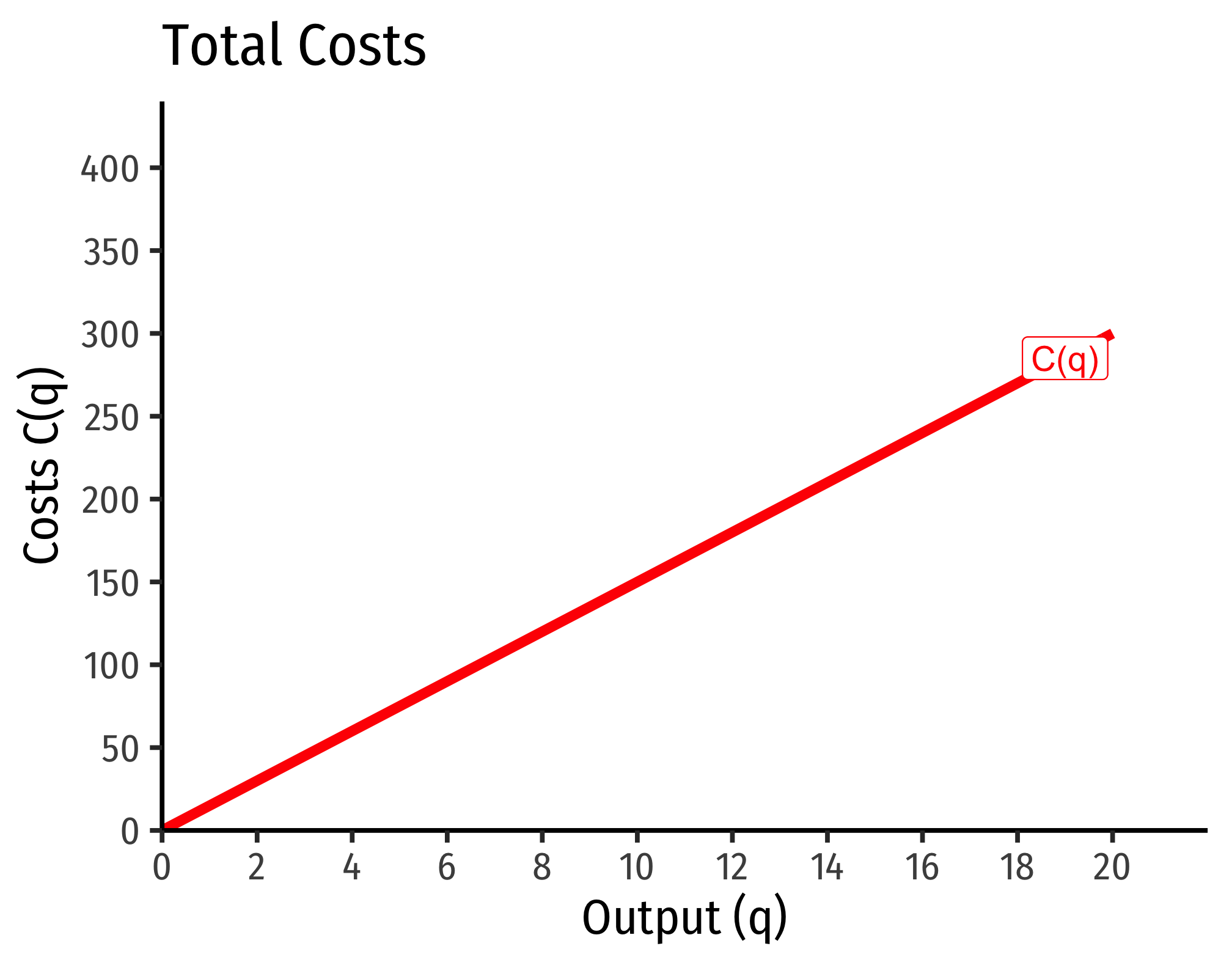Marginal costs would be

$MC(q) = \frac{\partial C(q)}{\partial q} = 15$

Average costs would be

$MC(q) = \frac{C(q)}{q} = \frac{15q}{q} = 15$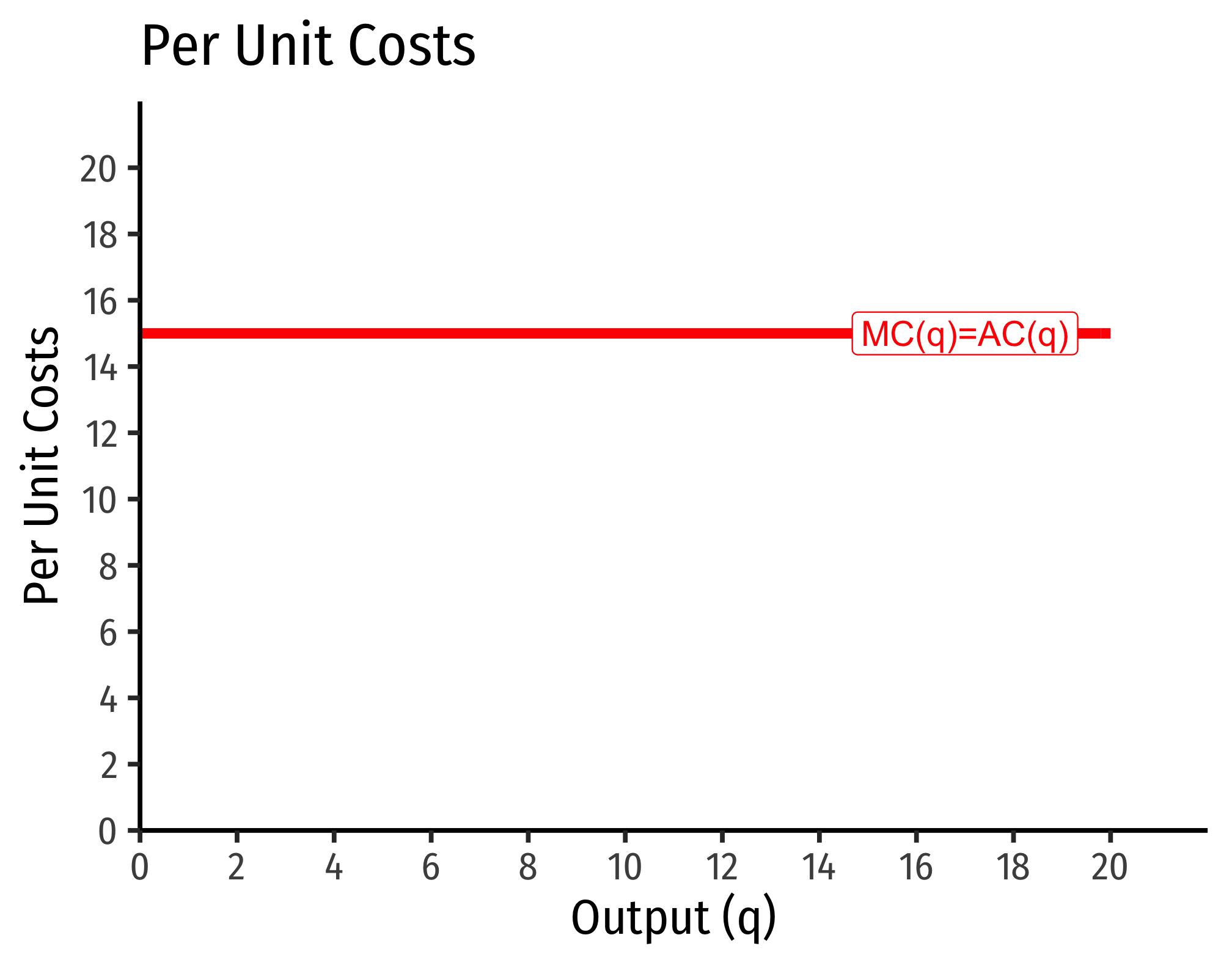#### Example (Decreasing Returns)

Let $$q=l^{0.25}k^{0.25}$$.

\begin{align*} C(w,r,q)&=\left[\left(\frac{0.25}{0.25}\right)^{\frac{0.25}{0.25+0.25}} + \left(\frac{0.25}{0.25}\right)^{\frac{-0.25}{0.25+0.25}}\right] w^{\frac{0.25}{0.25+0.25}} r^{\frac{0.25}{0.25+0.25}} q^{\frac{1}{0.25+0.25}}\\ C(w,r,q)&= \left[1^{0.5}+1^{-0.5} \right]w^{0.5}r^{0.5}q^{2}\\ C(w,r,q)&= w^{0.5}r^{0.5}q^{2}\\ \end{align*}

If $$w=9$$, $$r=25$$:

\begin{align*}C(w=10,r=20,q)&=9^{0.5}25^{0.5}q^2 \\ & =3*5*q^2\\ & =15q^2\\\end{align*}Marginal costs would be

$MC(q) = \frac{\partial C(q)}{\partial q} = 30q$

Average costs would be

$AC(q) = \frac{C(q)}{q} = \frac{15q^2}{q} = 15q$